# 1. 文章贡献

1. 本文提出了一种称为扫描比测试(SRT)的快速而健壮的方法，基于城市环境中大多数动态对象(如地面车辆和行人)不可避免地与地面接触的性质，获取包含动态点的栅格。
2. 获取栅格后，我们应用区域地平面拟合(R-GPF)，这是一种计算负荷低的新型静态点检索方法。R-GPF克服了基于射线跟踪的方法和基于可见性的方法的潜在局限性，如图2所示。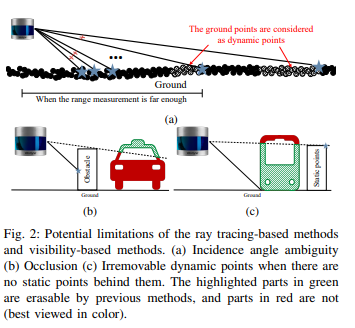3. 与其他最先进的方法进行验证。本文指出了精度/召回指标的局限性，并提出了适用于静态地图构建任务的替代指标:保存率和拒绝率。

4. 本文提出的方法比最先进的方法显示出有前途的性能。特别是，ERASOR以最小的静态点损失去除动态点，比目前最先进的方法快至少10倍。

# 2. 详细内容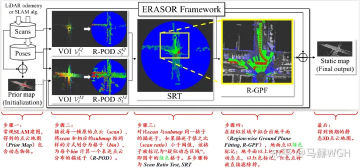)

### 2.1 问题定义与符号系统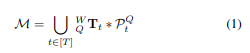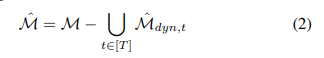ERASOR 的策略中的\hat{M}_{dyn,t}指由P^Q_tP^M_t之间的差异，并将P^M_t中动态的点云并标记出来。

1. 在$$P^M_t$$中有一个物体在地面上，而在$$P^Q_t$$中相同位置的地面不受物体的影响。
2. 在$$P^M_t$$中，地面不受物体的影响，而在$$P^Q_t$$中，地面上有相同位置的物体。
3. $$P^M_t$$和$$P^Q_t$$中都有一个物体在地面上。
4. 在$$P^M_t$$和$$P^Q_t$$中地面上没有物体。

### 2.2 栅格划分与占据状态描述子计算（R-POD）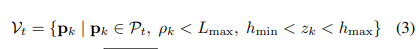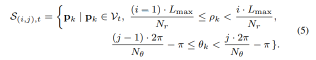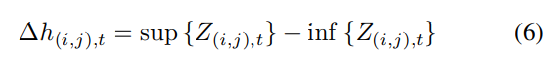)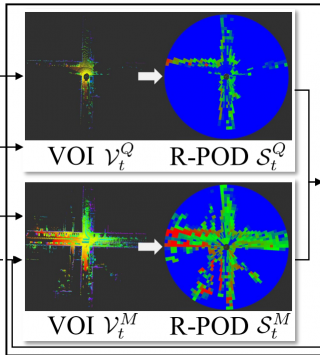### 2.3 根据描述子对比筛选潜在动态区域（SRT）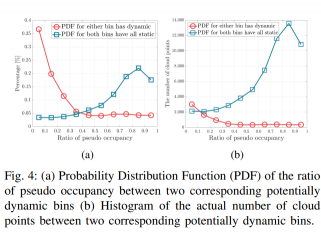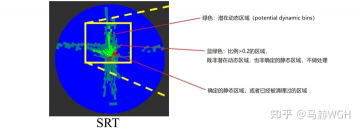### 2.4 地面拟合&动态点滤除（R-GPF）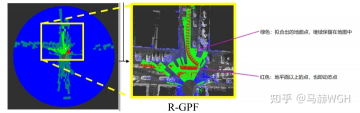# 3. 参考链接

https://zhuanlan.zhihu.com/p/409884870